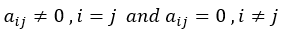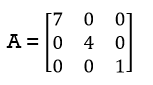Goseeko blog# What are matrices and their types?

Matrices have a wide range of applications in various disciplines such as chemistry, Biology, Engineering, Statistics, economics, etc. Matrices play an important role in computer science also. We widely use matrices to solve the system of linear equations, system of linear differential equations and nonlinear differential equations. First time the matrices were introduced by Cayley in 1860.

## Definition

A matrix is a rectangular arrangement of the numbers. We call these numbers inside the matrix as elements of the matrix.

HereThe expression of a matrix ‘A’ is-

The vertical elements are columns and the horizontal elements are rows of the matrix.

The order of matrix A is m by n or (m× n)

## Notation of a matrix

We denote a matrix ‘A’ is as-

Where, i = 1, 2, …….,m and j = 1,2,3,…….n

Here ‘i’ denotes row and ‘j’ denotes column.

## Types of matrices

1. Rectangular matrix-

A matrix in which the number of rows is not equal to the number of columns, then we say that the given matrix is a rectangular matrix.

Example:

The order of matrix A is 2×3, hence, it has two rows and three columns.

Matrix A is a rectangular matrix.

2. Square matrix-

A matrix with equal number of rows and columns, is a square matrix.

Example:

The order of matrix A is 3 ×3 , that means it has three rows and three columns.

Matrix A is a square matrix.

3. Row matrix-

A matrix with a single row and any number of columns is called row matrix.

Example:

4. Column matrix-

A matrix with a single column and any number of rows is called row matrix.

Example:

5. Null matrix (Zero matrix)-

A matrix in which each element is zero, then we call it a null matrix or zero matrix, therefore, we denote it by O.

Example:

6. Diagonal matrix-

A matrix is said to be diagonal matrix if all the elements except principal diagonal are zero

therefore, The diagonal matrix always follows-So that, for Example:7. Scalar matrix-

A diagonal matrix in which all the diagonal elements are equal to a scalar, hence, we call it a scalar matrix.

Example-

8. Identity matrix-

A diagonal matrix is said to be an identity matrix if its each element of diagonal is unity or 1.

Hence, we denote it by – ‘I’

9. Triangular matrix-

If every element above or below the leading diagonal of a square matrix is zero. therefore, we call it a triangular matrix.

Hence, there are two types of triangular matrices-

(a) Lower triangular matrix-

If all the elements below the leading diagonal of a square matrix are zero, therefore, we call it a lower triangular matrix.

Example:

(b) Upper triangular matrix-

If all the elements above the leading diagonal of a square matrix are zero, hence, we call it a lower triangular matrix.

Example –